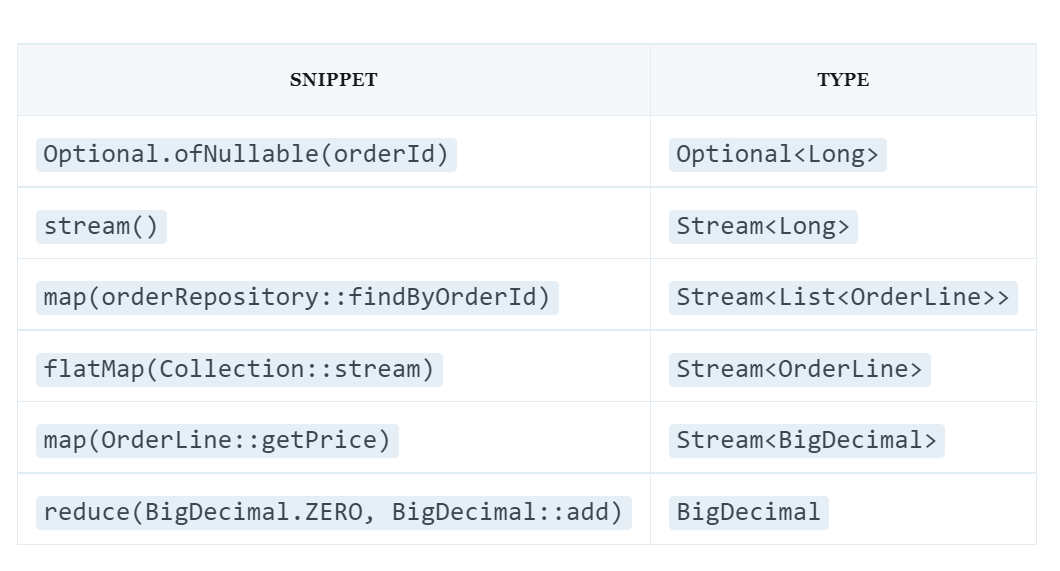# Optional.stream ()This week I learned about an interesting “new” feature of Optional that I want to talk about in this post. It has been available since Java 9, so the newness is relative.

Let’s start with the following sequence for calculating the total order price:

``````public BigDecimal getOrderPrice(Long orderId) {
List<OrderLine> lines = orderRepository.findByOrderId(orderId);
BigDecimal price = BigDecimal.ZERO;
for (OrderLine line : lines) {
price = price.add(line.getPrice());
}
return price;
}``````
• Provide variable-accumulator for price

• Add the price of each line to the total price

Nowadays, it is probably more appropriate to use streams instead of iterations. The following snippet is equivalent to the previous one:

``````public BigDecimal getOrderPrice(Long orderId) {
List<OrderLine> lines = orderRepository.findByOrderId(orderId);
return lines.stream()
.map(OrderLine::getPrice)
.reduce(BigDecimal.ZERO, BigDecimal::add);
}``````

Let’s focus on the variable `orderId` : it can contain `null`

Imperative processing method `null` is to test it at the beginning of the method – and eventually reset:

``````public BigDecimal getOrderPrice(Long orderId) {
if (orderId == null) {
throw new IllegalArgumentException("Order ID cannot be null");
}
List<OrderLine> lines = orderRepository.findByOrderId(orderId);
return lines.stream()
.map(OrderLine::getPrice)
.reduce(BigDecimal.ZERO, BigDecimal::add);
}``````

The functional way is to wrap `orderId` in Optional. This is what the code looks like using Optional:

``````public BigDecimal getOrderPrice(Long orderId) {
return Optional.ofNullable(orderId)
.map(orderRepository::findByOrderId)
.flatMap(lines -> {
BigDecimal sum = lines.stream()
.map(OrderLine::getPrice)
.reduce(BigDecimal.ZERO, BigDecimal::add);
return Optional.of(sum);
}).orElse(BigDecimal.ZERO);
}``````
1. Wrap `orderId` in `Optional`

2. Find the relevant order lines

3. Use `flatMap()`, To obtain `Optional<BigDecimal>`; `map()` will receive `Optional<Optional<BigDecimal>>`

4. We need to wrap the result in `Optional`to match the method signature.

5. If a `Optional` contains no value, sum is `0`

`Optional` makes the code less readable! I believe that clarity should always be more important than code style.

Fortunately, `Optional` suggests a method `stream()` (since Java 9). It allows you to simplify the functional pipeline:

``````public BigDecimal getOrderPrice(Long orderId) {
return Optional.ofNullable(orderId)
.stream()
.map(orderRepository::findByOrderId)
.flatMap(Collection::stream)
.map(OrderLine::getPrice)
.reduce(BigDecimal.ZERO, BigDecimal::add);
}``````

Here is a summary of the type on each line:Functional code does not necessarily mean that it is also readable code. Given the recent changes, I believe it is both.

Translation of the material prepared as part of the course “Java Developer. Basic”… We invite everyone to Open Day online, where you can learn more about the format and program of training, as well as get to know the teacher.